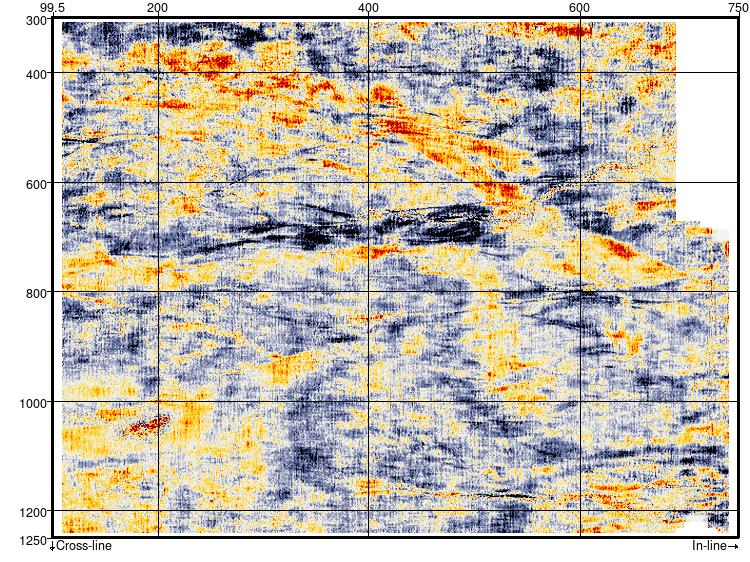Script: Filtering/ex_spatial_filter_rectangular.py

### Description

This Python ExternalAttrib script applies lowpass, highpass, bandpass or band reject spatial filters with rectangular symmetry. The filters are applied by direct spatial convolution of a kernel formed by cascading two 1D MAXFLAT operators (Khan and Ohba (2001)).

By setting the stepout in one direction to 0 the filter will be applied as a 1D spatial filter in the other direction.

Note that the filter cutoff or band pass/reject frequency is specified in Normalised Spatial Frequency. Normalised Frequency ranges from 0 to 1 at the spatial nyquist. Converting a spatial frequency in cycles/metre to the equivalent Normalised Spatial Frequency is as simple as dividing it by the spatial nyquist frequency.

### Examples

This example shows inline and crossline FK spectra after applying a 2D lowpass rectangular filter with inline and crossline normalised frequency cutoffs of 0.6 and 0.3 respectively.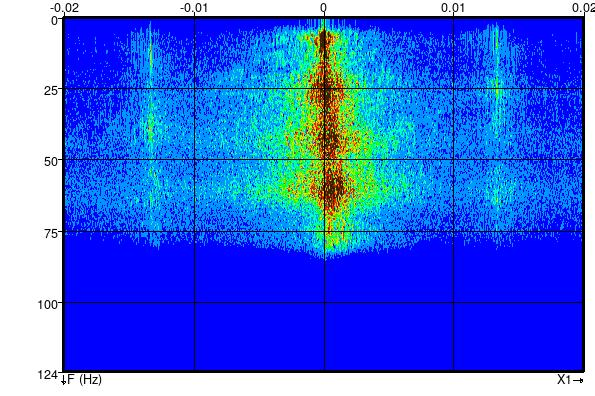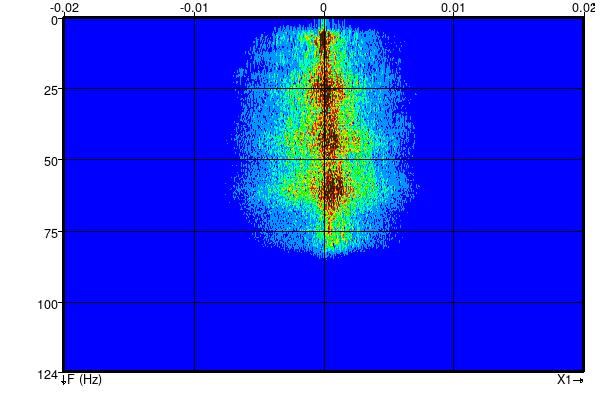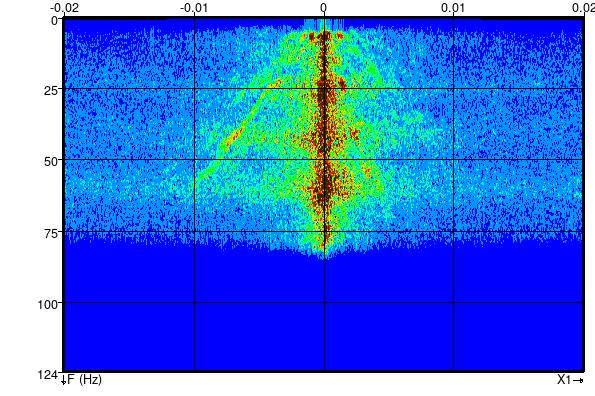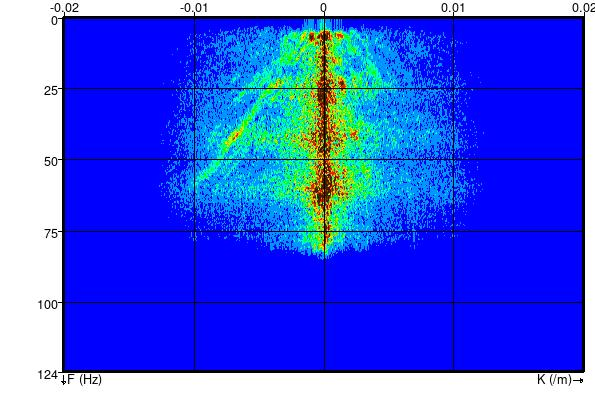This example shows a timeslice at 300ms TWT from the F3 Demo dataset after applying a 1D spatial filter along the inlines (stepout of 0,9) with a crossline normalised frequency cutoff of 0.5.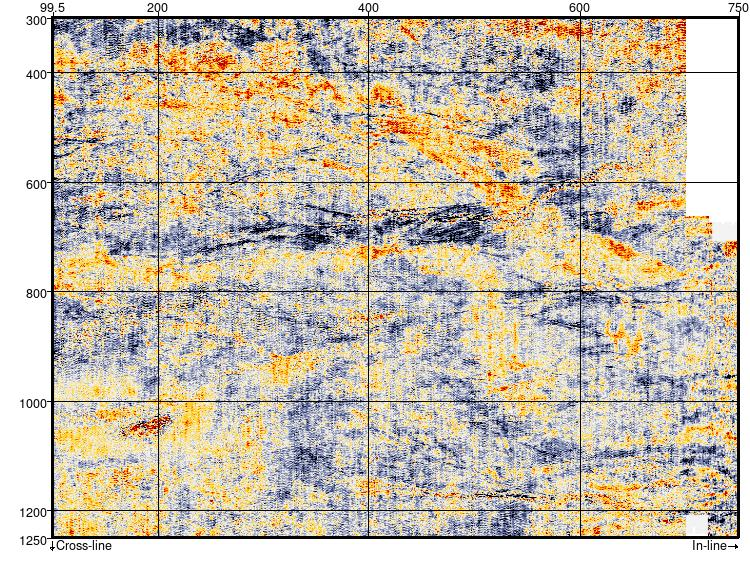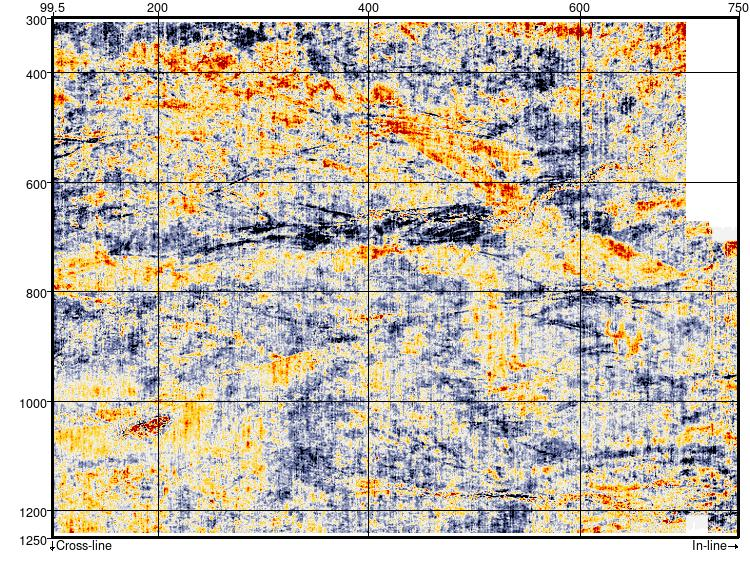This example shows the timeslice at 300ms TWT from the F3 Demo dataset with the crossline lowpass filter above followed by a 1D bandreject spatial filter along the crosslines (stepout 9,0) with an inline normalised rejection frequency of 0.17.